Скачать презентацию Section 3 6 1 Implicitly differentiate to find

0791b705831e286dee229e996b226bc5.ppt

• Количество слайдов: 16Section 3. 6 1. Implicitly differentiate to find dy/dx. y 3 – x 2 = 4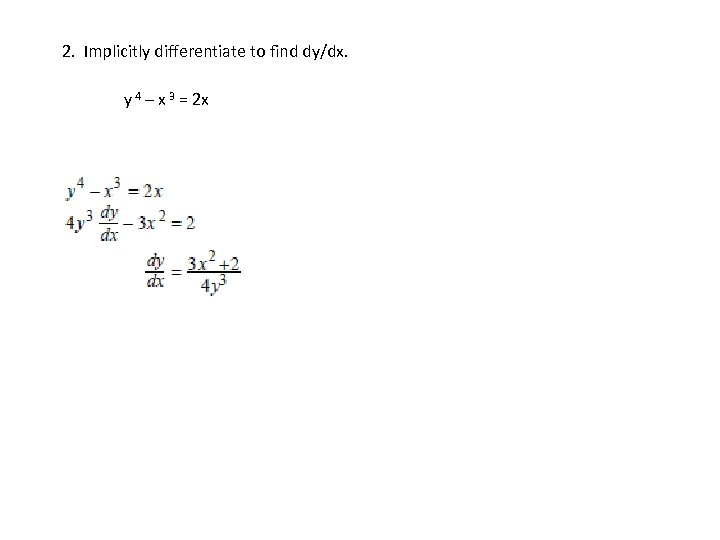2. Implicitly differentiate to find dy/dx. y 4 – x 3 = 2 x3. Implicitly differentiate to find dy/dx. x 2 y = 8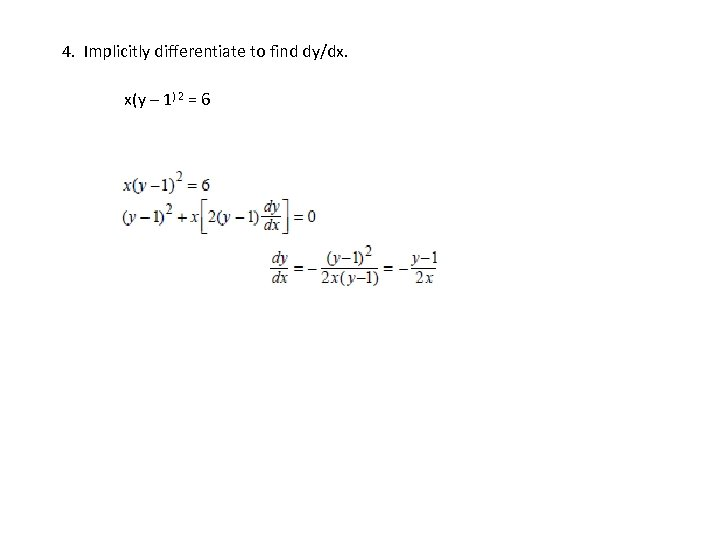4. Implicitly differentiate to find dy/dx. x(y – 1) 2 = 6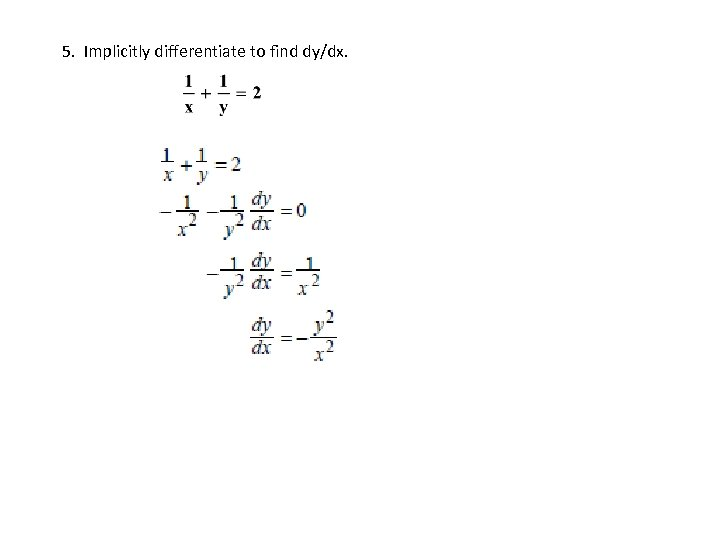5. Implicitly differentiate to find dy/dx.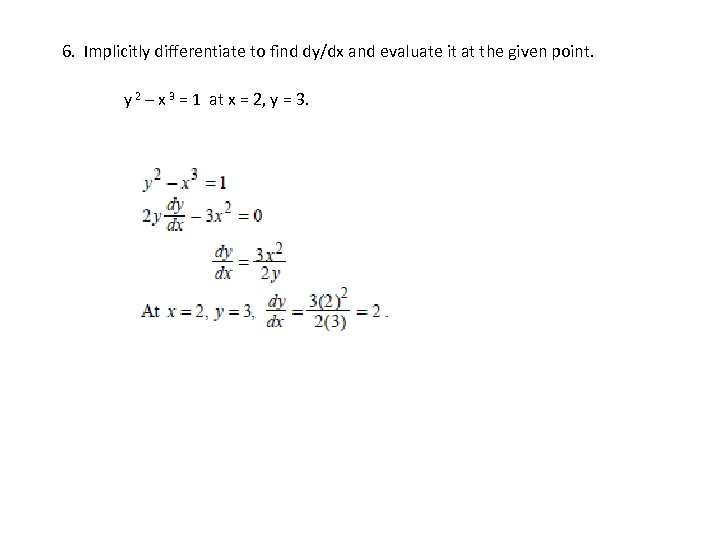6. Implicitly differentiate to find dy/dx and evaluate it at the given point. y 2 – x 3 = 1 at x = 2, y = 3.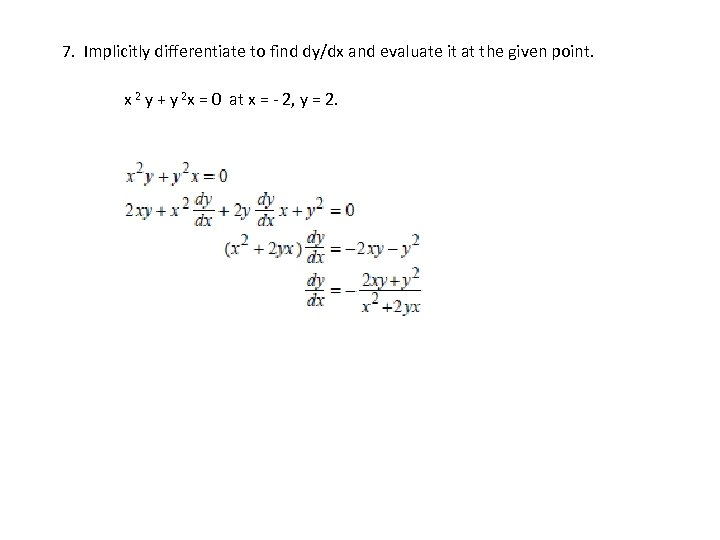7. Implicitly differentiate to find dy/dx and evaluate it at the given point. x 2 y + y 2 x = 0 at x = - 2, y = 2.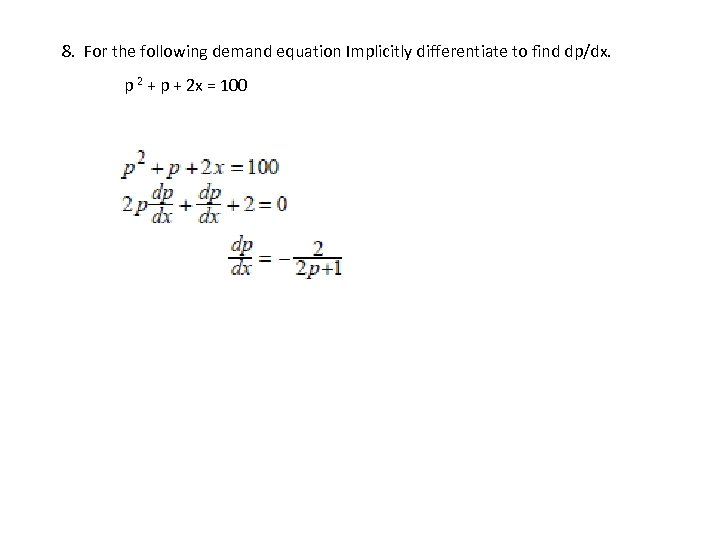8. For the following demand equation Implicitly differentiate to find dp/dx. p 2 + p + 2 x = 1009. For the following demand equation Implicitly differentiate to find dp/dx. xp 3 = 36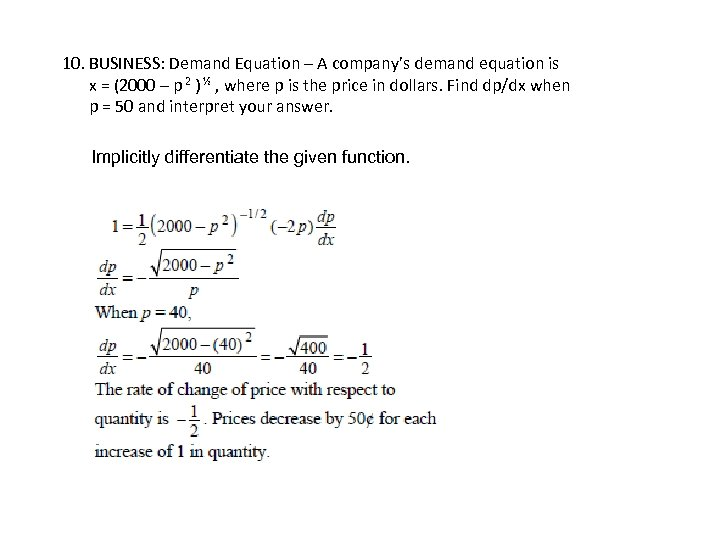10. BUSINESS: Demand Equation – A company’s demand equation is x = (2000 – p 2 ) ½ , where p is the price in dollars. Find dp/dx when p = 50 and interpret your answer. Implicitly differentiate the given function.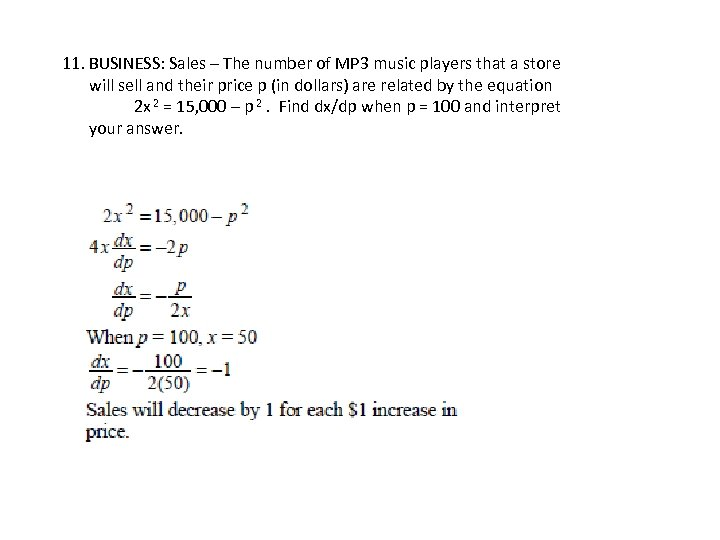11. BUSINESS: Sales – The number of MP 3 music players that a store will sell and their price p (in dollars) are related by the equation 2 x 2 = 15, 000 – p 2. Find dx/dp when p = 100 and interpret your answer.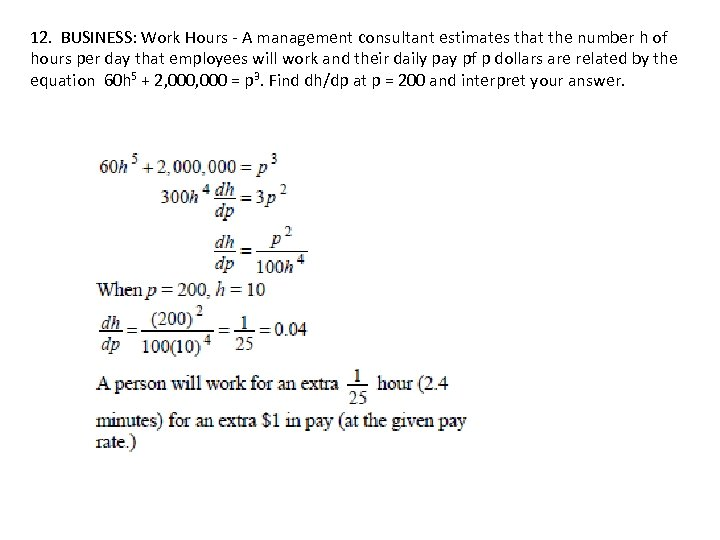12. BUSINESS: Work Hours - A management consultant estimates that the number h of hours per day that employees will work and their daily pay pf p dollars are related by the equation 60 h 5 + 2, 000 = p 3. Find dh/dp at p = 200 and interpret your answer.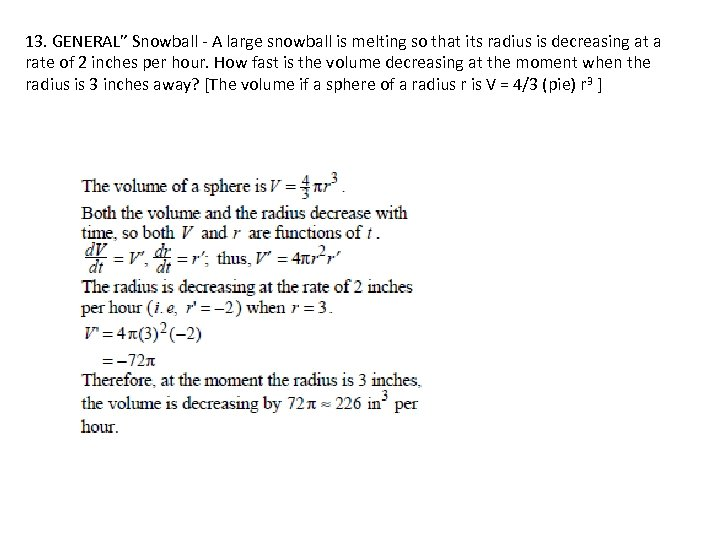13. GENERAL” Snowball - A large snowball is melting so that its radius is decreasing at a rate of 2 inches per hour. How fast is the volume decreasing at the moment when the radius is 3 inches away? [The volume if a sphere of a radius r is V = 4/3 (pie) r 3 ]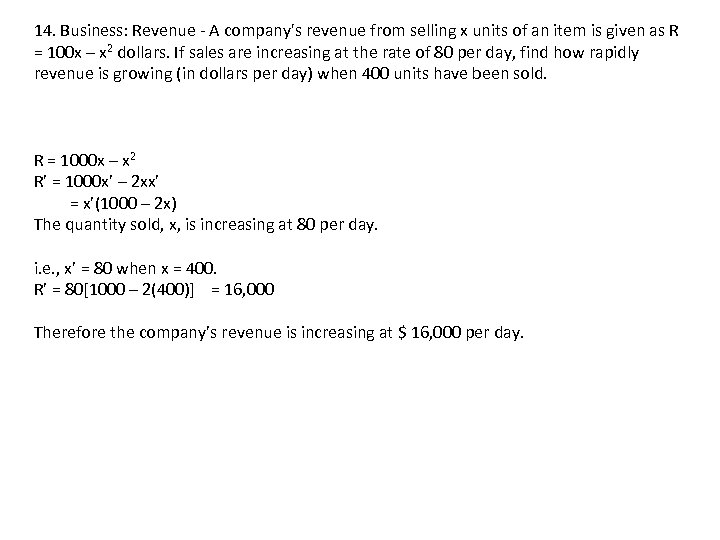14. Business: Revenue - A company's revenue from selling x units of an item is given as R = 100 x – x 2 dollars. If sales are increasing at the rate of 80 per day, find how rapidly revenue is growing (in dollars per day) when 400 units have been sold. R = 1000 x – x 2 R’ = 1000 x’ – 2 xx’ = x’(1000 – 2 x) The quantity sold, x, is increasing at 80 per day. i. e. , x’ = 80 when x = 400. R’ = 80[1000 – 2(400)] = 16, 000 Therefore the company’s revenue is increasing at \$ 16, 000 per day.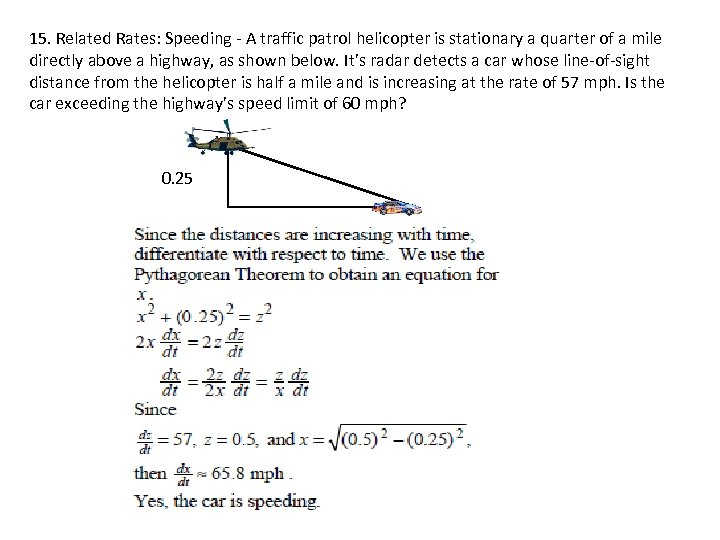15. Related Rates: Speeding - A traffic patrol helicopter is stationary a quarter of a mile directly above a highway, as shown below. It’s radar detects a car whose line-of-sight distance from the helicopter is half a mile and is increasing at the rate of 57 mph. Is the car exceeding the highway’s speed limit of 60 mph? 0. 25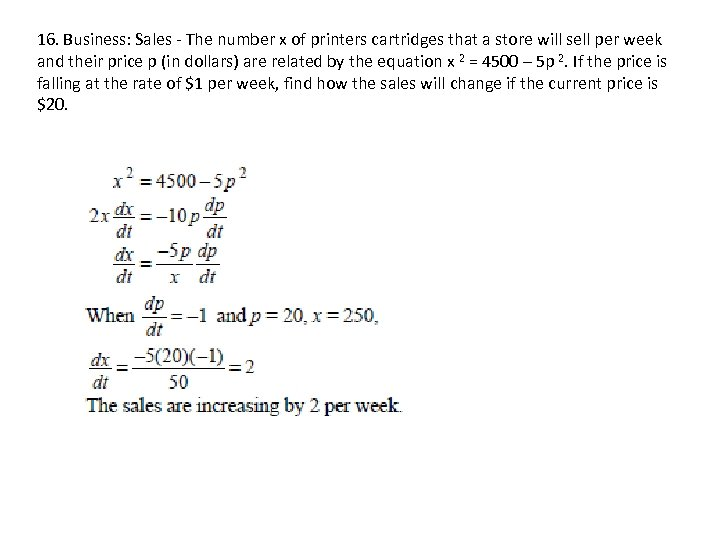16. Business: Sales - The number x of printers cartridges that a store will sell per week and their price p (in dollars) are related by the equation x 2 = 4500 – 5 p 2. If the price is falling at the rate of \$1 per week, find how the sales will change if the current price is \$20.